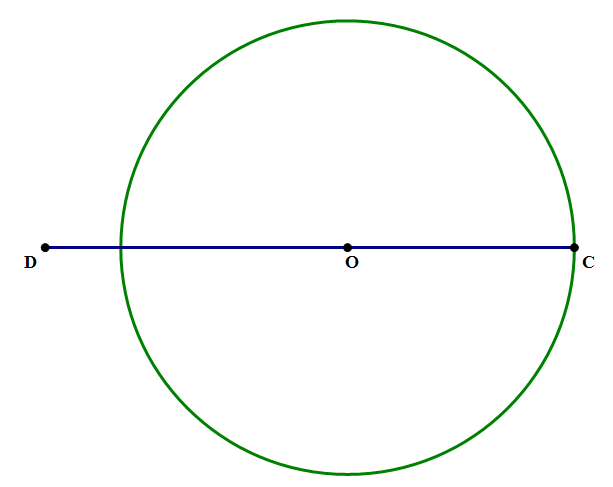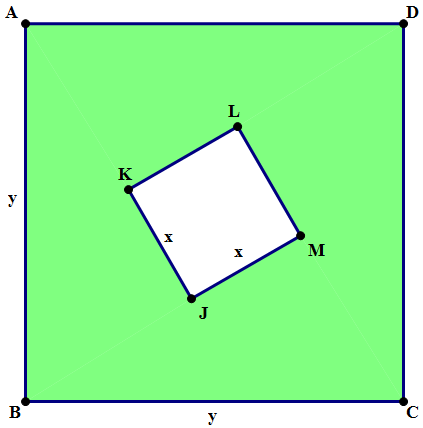# Three Essential Algebra Formulas

### First, a few practice problems.

1. The numbers a, b, and c are all positive. If \space{b}^2 + {c}^2 = 117, then what is the value of {a}^2 + {c}^2?

Statement #1: a - b = 3

Statement #2: \frac{(a + b)}{(a - b)} = 7

2. Given that (P + 2Q) is a positive number, what is the value of (P + 2Q)?

Statement #1: Q = 2

Statement #2: {P}^2 + 4PQ + 4{Q}^2 = 283. In the diagram above, O is the center of the circle, DC = a and DO = b. What is the area of the circle?

Statement #1: {a}^2 - 2ab + {b}^2 = 36

Statement #2: a + b = 224. ABCD is a square with a side y, and JKLM is a side x. If Rectangle S (not shown) with length (x + y) has the same area as the shaded region above, what is the width of Rectangle S?

(A) x

(B) y

(C) y + x

(D) y - x

(E) {y}^2 - {x}^2

### Three important algebra patterns

Doing math involve both following procedures and recognizing patterns. Three important patterns for algebra on the QUANT are as follows:

Pattern #1: The Difference of Two Squares

{A}^2 - {B}^2 = (A + B)(A - B)

Pattern #2: The Squares of a Sum

{(A + B)}^2 = {A}^2 + 2AB + {B}^2

Pattern #3: The Squares of a Difference

{(A - B)}^2 = {A}^2 - 2AB + {B}^2

For Quant success, you need to know these patterns cold. You need to know them as well as you know your own phone number or address. The test will throw question after question at you in which you simply will be expected to recognize these patterns. In such a question, if you recognize the relevant formula, it will enormously simplify the problem. If you don’t recognize the relevant formula, you are likely to be stymied by such a question.

### Memory, not memorizing

You might think I would say: memorize them. Instead, I will ask you to remember them. What’s the difference? Memorization implies a rote process, simply trying to stuff an isolated and disconnected factoid into your head. By contrast, you strengthen you capacity to remember a math formula when you understand all the logic that underlies it.

Here, the logic behind these formulas is the logic of FOILing and factoring. You should review those patterns until you can follow each both ways - until you can FOIL the product out, or factor it back into components. If you can do that, you really understand these, and are much more likely to remember them in an integrated way.

### Summary

If these patterns are relatively new to you, you may want to revisit the problems at the top with the list handy: see if you can reason your way through them, before reading the explanations below.

### Practice Problem Explanations

1. Let X = a^2 + c^2, the value we are seeking. Notice if we subtract the first equation in the prompt from this equation, we get a^2 - b^2 = X - 117. In other words, if we could find the value of a^2 - b^2, then we could find the value of X.

Statement #1: a - b = 3

From this statement alone, we cannot calculate a^2 - b^2, so we can’t find the value of X. Statement #1, alone and by itself, is insufficient.

Statement #2: \frac{(a + b)}{(a - b)} = 7

From this statement alone, we cannot calculate a^2 - b^2, so we can’t find the value of X. Statement #2, alone and by itself, is insufficient.

Statements #1 & #2 combined: Now, if we know both statements are true, then we could multiple these two equations, which cancel the denominator, and result in the simple equation a + b = 21. Now, we have the numerical value of both (a - b) and (a + b), so from the difference of two squares formula, we can figure out a^2 - b^2, and if we know the numerical value of that, we can calculate X and answer the prompt. Combined, the statements are sufficient.

2. The prompt tells us that (P + 2Q) is a positive number, and we want to know the value of P. Remember number properties! We don’t know that (P + 2Q) is a positive integer, just a positive number of some kind.

Statement #1: Q = 2

Obvious, by itself, this tells us zilch about P. Alone and by itself, this statement is completely insufficient.

Statement #2: P^2 + 4PQ + 4Q^2 = 28

Now, this may be a pattern-recognition stretch for some folks, but this is simply the “Square of a Sum” pattern. It may be clearer if we re-write it like this:

P^2 + 2*P*(2Q) + (2Q)^2 = 28

This is now the “Square of a Sum” pattern, with P in the role of A and 2Q in the role of B. Of course, this should equal the square of the sum:

P^2 + 2*P*(2Q) + (2Q)^2 = (P + 2Q)^2 = 28

All we have to do is take a square root. Normally, we would have to consider both the positive and the negative square root, but since the prompt guarantees that (P + 2Q) is a positive number, we need only consider the positive root:

(P + 2Q) = \sqrt{28}

This statement allows us to determine the unique value of (P + 2Q), so this statement, alone and by itself, is sufficient.

3. To find the area of the circle, we need to use Archimedes’ formula, A = \pi(r^2). For that we need the radius, OC. We are not given this directly, but notice that r = OC = DC - OD = a - b. If we knew that, we could find the area of the circle.

Statement #1: a^2 - 2ab + b^2 = 36

A major pattern-matching hit! This, as written, is the “Square of a Difference” pattern.

a^2 - 2ab + b^2 = (a - b)^2 = r^2= 36

In fact, this statement already gives us r^2, so we just have to multiply by pi and we have the area. This statement, alone and by itself, is sufficient.

Statement #2: a + b = 22

We need a - b, and this statement gives us a value of a + b. If we had more information, perhaps we could use this in combination with other information to find what we want, but since this is all we have, it’s simply not enough to find a - b. This statement, alone and by itself, is insufficient.